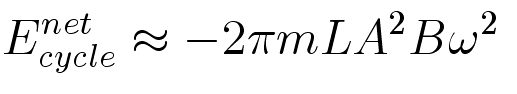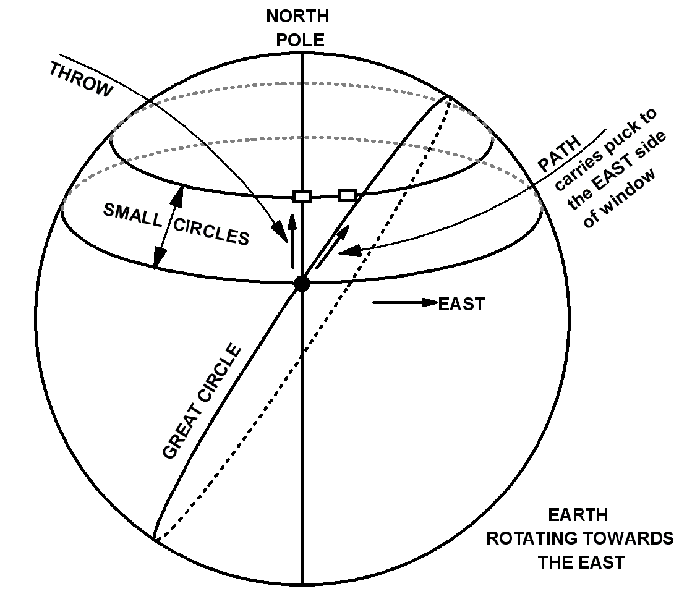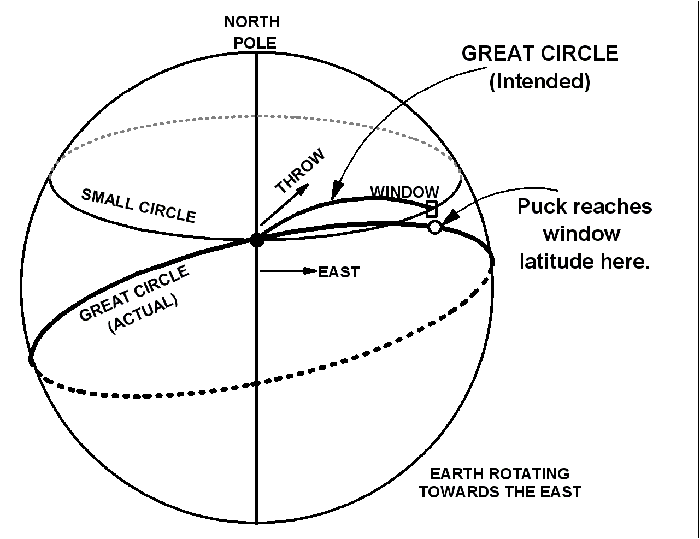# Experiment of The Month

## Pendulum Celebration

Interest in the Foucault pendulum leads to this strategy of using the pendulum through an introductory college mechanics course. The overall strategy is to have the Foucault pendulum running all the time, “in background.” Attention is called to it during the normal development of the course, when applications are appropriate. At some point students will comment on the fact that the plane of oscillation changes. At this point the Foucault pendulum and the rotation of the earth can be discussed. A version of this discussion is at the end of the document.

##### Position, Velocity And Acceleration

Since the motion is along a circular arc, it is easiest to discuss tangential component of the motion. This motion offers examples of velocity and acceleration that differ from projectile motion. In particular, the acceleration is not constant.

• The tangential position (measured from the low point of the swing) changes, up to a maximum, the amplitude of the motion.
• The tangential velocity is zero when position is maximum and greatest at the low point. This is similar to vertical projectile motion, but not the same, because acceleration is not constant.
• It is useful to think of the motion as starting from rest at the maximum position and accelerating downward along the curve.
• The tangential acceleration is greatest when position is maximum and zero at the low point.
##### Circular Motion

The centripetal acceleration is v2/R even if the magnitude of the velocity is not constant.

• The radial position is constant and the radial velocity is zero.
• The radial acceleration is the centripetal acceleration; maximum at the low point of the swing and zero at the top of the swing.
##### Newton's Laws

The undriven pendulum involves two force vectors, by the string and by gravity, adding to cause the pendulum acceleration.

• The net force is a vector sum that lies in the plane defined by the two individual force vectors.
• The acceleration is the same direction as the net force.
• Even though the string tension and acceleration change, the motion remains in the plane defined by the string force and the gravity force.
• The net force has both radial and tangential components, as does the acceleration.
##### Friction And Drag Forces

With the driver turned off, the pendulum dies down. A plot of pendulum amplitude versus time suggests an exponential decay, consistent with a drag force proportional to velocity through the air. Closer examination shows significant deviation from exponential behavior.

##### Work And Energy
• Energy in an ideal pendulum flows back and forth between kinetic energy and potential energy, with total energy staying constant.
• In the real pendulum, friction converts some of the total mechanical energy to heat each cycle. The friction work equals the loss in mechanical energy.
• The loudspeaker pump adds potential energy to the pendulum at the bottom of the swing by doing work.
• The displacement is nearly vertical while, the bob velocity is nearly horizontal. To a fair approximation, at the bottom of the swing, the speaker work has little direct effect on the kinetic energy.
• At the top of the swing, the loudspeaker moves back down. Because the string only pulls and cannot push, the loudspeaker does no work on the pendulum during this motion.
• The top of the swing is higher because the bob reached it with the speaker in its “up” position. The bob swings downward with the speaker in its “down” position.
• The drop in potential energy is larger than in would have been if the speaker had not acted. The resulting kinetic energy at the bottom of the swing is larger than it would have been if the speaker had not acted.
• The speaker adds a fixed amount of potential energy at the bottom of the swing.

(Aside: Meron Wollie calculated the total energy added per cycle to bewhere m is the bob mass, L is the string length, A is the angular pendulum amplitude, B is the loudspeaker amplitude, and omega is the angular frequency. Her results may be viewed in the archive )

• If the energy added by the speaker is greater than the energy lost to friction, the pendulum amplitude increases. When this happens the friction loss increases, and eventually the pendulum finds a steady state amplitude.
• If the speaker moves down when the pendulum is at the bottom of the swing, it removes energy from the swing, “un-pumping” the motion until the pendulum stops swinging.
##### Non-inertial Frame Of Reference

The driver may be considered as an application of artificial gravity in a non-inertial frame of reference:

• In a frame of reference that moves with the loudspeaker driver, the speaker is stationary, and the lab moves up and down. This is an accelerated, non-inertial frame of reference.
• An accelerated frame of reference displays an “artificial gravity” in addition to “normal” gravity. The size of the artificial gravity is the same as the size of the acceleration, and the direction is opposite to the acceleration direction.
• In the non-inertial frame, “gravity” is larger when the speaker accelerates up, and smaller when the speaker accelerates down.
• The speaker pulls up just before the low point, so there is an extra gravity force adding speed to the pendulum.
• The speaker drops down just before the high point, so that the pendulum swings higher in the lower gravity.
##### Momentum

Neither linear nor angular momentum is conserved for the pendulum, as evidenced by the fact that the bob momentum is zero twice each period.

##### Rotational Motion

The equations of motion for the pendulum are compactly expressed in terms of torque, moment of inertia and angular momentum.

##### Simple Harmonic Motion
• The standard simple harmonic motion discussion may be applied to the pendulum, short term, with the driver off, for small amplitude.
• Long term, with the driver turned off, the pendulum must be described in terms of damped harmonic motion. Exponential decay of the amplitude is easiest to analyze, but is only approximately true in the experiment.
• The “decay time” time for the amplitude to fall to approximately 1/3 (strictly 1/e) of its original amplitude is the inverse of the width of the resonance curve.
• With the driver on, the motion is driven damped harmonic motion. The driver frequency must match the natural frequency in order to maintain a long term effect.
• The driver will be effective its frequency is within the width of the resonance curve. It will work whether the driver frequency is above or below the natural frequency.
• In real life, the pendulum natural frequency is somewhat lower for larger amplitudes. The best driver frequency is slightly above the natural frequency of the pendulum. That way, if the amplitude increases, the effectiveness of the driver decreases. This negative feedback decreases the amplitude back towards it nominal value. Driving above the resonance increases the stability of the system.
##### Earth In Motion

The rotation of the plane of oscillation of the Foucault pendulum is caused by the rotation of the earth on its axis. This is most easily understood by imagining a pendulum with its pivot point above the north pole. The pendulum swings in an unchanging plane of oscillation while the earth rotates (to the east, counterclockwise when looking down from above the north pole) around it once per day. From the point of view of a passenger on the earth, the pendulum rotates clockwise once per day.

For locations not at the pole, the argument requires more detail. A mathematical discussion is available here: corirot.htm

For a pictorial discussion, see the following.

##### Sliding A Puck North On A Spinning EarthWe use a hockey puck as a surrogate for the pendulum bob because the motion of the puck is not accelerated (assuming frictionless ice). The puck, constrained to slide on the surface of the earth follows a great circle path, as sketched.

Imagine sliding a puck towards the window of the hockey goal. If the earth does not spin, the great circle followed by the puck is a meridian; a line of longitude going through the north pole. That line is represented by a vertical line in the drawing.

If the earth spins, rotating towards the east, then in addition to the northward velocity component, the puck has an eastward component. It follows the “tilted” great circle in the figure. The window of the goal also moves to the east.

However, the window moves slower, since in one day in travels the circumference of a smaller circle than the starting point of the puck. This means that the eastward component of motion of the puck is greater than the window, and the puck misses, passing to the east of the window.

If the puck were launched toward a window that was to the south, the window would have a larger eastward velocity, and the puck would miss to the west. The pendulum rotates clockwise with each swing.

##### Sliding A Puck East On A Spinning EarthThis time we want to send the puck to a window that is due east of us. We know that the puck will follow a great circle, and that only one great circle passes between the launch point and also the window. We determine that great circle with the assumption that the earth is stationary. The predicted path for the puck is sketched as a heavy line from start to window in the figure above.

We throw the puck along the direction of the great circle. Because the earth is rotating east, the puck velocity has an additional eastward component added to our throwing velocity vector. The result is that the puck is launched at too shallow an angle. It follows a different great circle, which carries it to the south side of the window.

If the window was to the west of us, our launch angle would be too great, and the different great circle would rise too high, carrying the puck to the north side of the window.

The pendulum similarly rotates clockwise with each swing.

Because the pendulum plane rotates clockwise with each swing, the rotations accumulate enough that we can discern them much more clearly than we can see the rotation in a single swing.

Formal analysis (see e.g. Classical Dynamics of Particles and Systems by Jerry B. Marion, Stephen T. Thornton) gives the period of rotation of the plane of oscillation, in terms of the latitude, L, Rotation rate = (sin L )x(15 degrees per hour)

At the north pole, L is 90 degrees and the pendulum rotates once per day. At latitude 40 degrees, the pendulum takes about 36 hours to make a complete rotation, turning at about 10 degrees per hour instead of 15 degrees per hour.

Marion and Thornton say that the rotation was observed as early as 1659 by Vincenzo Viviani, a student of Galileo’s. .

## Experiment Of The Month

View past labs experiments.